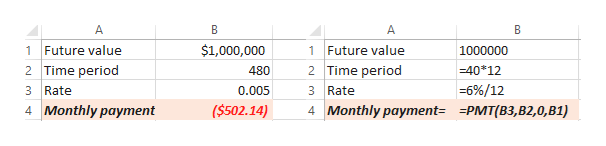# a. You are planning for retirement, and would like to have \$1,000,000 in the bank when you turn 65. (Assume that you just barely turned 25). How much should you deposit each month into an account earning 6% nominal interest per year, compounded monthly, to reach your goal? b. How much would you have to deposit each month, if the account earned 12% nominal interest per year, compounded monthly?

Question
3 views
a. You are planning for retirement, and would like to have \$1,000,000 in the bank when you turn 65. (Assume that you just barely turned 25). How much should you deposit each month into an account earning 6% nominal interest per year, compounded monthly, to reach your goal?

b. How much would you have to deposit each month, if the account earned 12% nominal interest per year, compounded monthly?
check_circle

Step 1

Compounding is a concept used in investing. In case of compounding, interest is earned on both the principal amount and the accumulated interest.

Step 2

Part a:
Given that:
The future value is \$1000000
Time period is the difference between 25 years and 65 years, that is equal to 40 years. As the compounding is happening monthly, the total number of compounding periods is 12 times 40 years, that is equal to 480 months.
Annual interest rate is 6%, we can get the monthly interest rate by dividing 6% by 12. So, the monthly interest rate is 0.005

We can determine the monthly payments using excel.

Step 3help_outlineImage TranscriptioncloseA B A B 1 Future value 2 Time period \$1,000,000 1 Future value 1000000 2 Time period 40*12 480 3 Rate 6%/12 0.005 3 Rate 4 Monthly payment (\$502.14) 4 Monthly payment= =PMT(B3,B2,0, B1) fullscreen

### Want to see the full answer?

See Solution

#### Want to see this answer and more?

Solutions are written by subject experts who are available 24/7. Questions are typically answered within 1 hour.*

See Solution
*Response times may vary by subject and question.
Tagged in